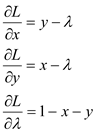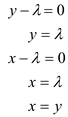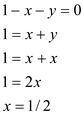# Quiz 2: Mathematics for Microeconomics

Utility function: It refers to a function use to measure the consumer's level of satisfaction derives from the consumption of goods and services. The utility function is given by,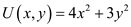a. Given the utility function, the partial differentiation with respect to a single variable, in a function of several variables is calculated as follows;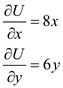b. Partial differentiation with respect to x is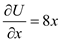and with respect to y is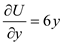. Compute partial derivatives at x= 1 and y = 2 by substituting the values of x and y in the above partial derivates computed in previous part. It is computed below: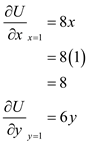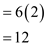c. Total differentiation is written with the help of partial differentiation.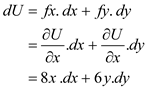d. Total differentiation is written with the help of partial differentiation.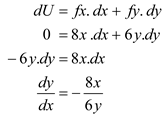So,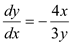It means trade-off between x and y is not linear. The negative sign shows that getting more x required forgoing some y and vice-versa. e. To prove that value of U is 16, when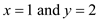, substitute the value of x and y in the utility function and solve as follows: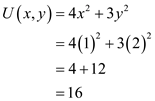Hence, the value of utility is 16. f. To determine the change in ratio of x and y while holding utility constant at 16, substitute the value of x and y in the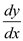computed in part d. It is computed below: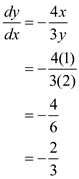Hence, (-2/3) ratio of x and y needed to hold U constant at 16. g. To plot the utility function, compute the horizontal and the vertical corner points as follows: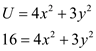Vertical points: When x is 0, then y will be,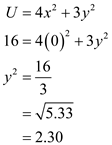Thus, vertical point is (0,2.30) Horizontal points: When y is 0, then x will be,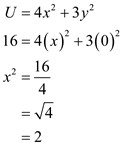Thus, horizontal point is (2,0). The following figure shows the shape of utility function when utility is 16: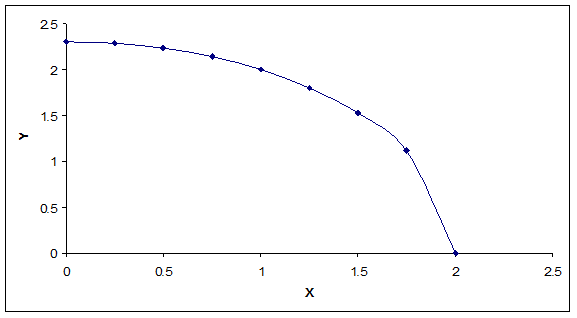As shown in the above figure, the shape of U is an ellipse centered at the origin. Slope of this equation is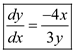.
Total revenue is the amount earned by the firm by its operations. Total cost is the production cost incurred by the firm to produce the commodity. Profit is the difference between the total revenue and total cost. a) Profit maximizing firm produces the output at that level where difference between total revenue and total cost is maximum.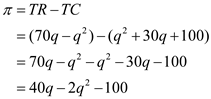Necessary condition is as follows: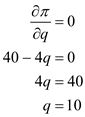Sufficient condition is as follows: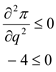Calculate the profit as follows: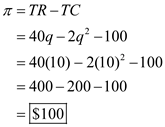Thus, firm produces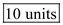of output and earns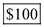profits. b) Second-order condition of profit maximization is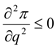. It is also called sufficient condition.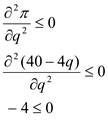Thus, second order condition is satisfied at 10 unit of output. c. Profit maximizes where marginal revenue is equal to marginal cost. Marginal revenue is additional revenue in total revenue while selling one more unit of product. Marginal cost is additional cost in total cost while producing one more unit of product. Calculate as follows: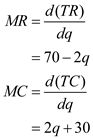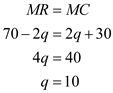Yes, the solution obeys the rule 'marginal revenue equals to marginal cost'.
Substitute goods are those good that can be used in place of other good and satisfaction level of both products is almost the same. Substitution method Sum of x and y is 1. Therefore, we can write it as:-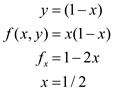Condition of consumer equilibrium (which seeks to solve the maximization and minimization problems subject to some constraints) can be easily understood with the help of Lagrange multiplier. Lagrange multiplier method:- Sum of x and y is 1. Budget constraint can be written as:-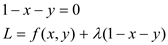Taking first order condition:-